# Rectangles versus rhombuses

 Page 2/2 Date 30.04.2018 Size 104.02 Kb. #42439
1   2

## 2. Rectangles versus rhombuses:

Which properties do they have in common?

Which properties distinguish rhombuses from rectangles (and vice-versa?)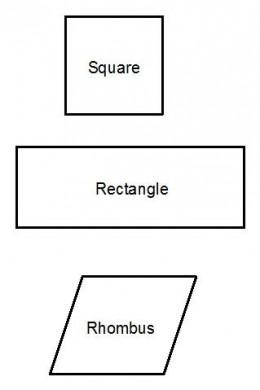## 3. Application of parallelograms: Adding Vectors.

The forces acting on an object determine the direction in which the object will move. A force is represented by a vector, showing not only how large the force is, but also the direction in which it acts.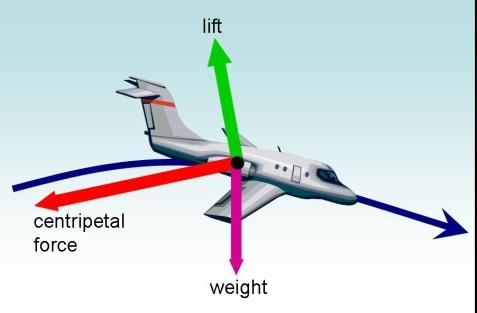Each vector is given by two plane coordinates and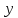. If two forces act on an object at the same time, then the direction in which the object will move will be given by the sum of the two vectors: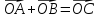. The vector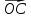is defined in coordinates by: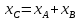and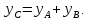Here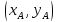are the coordinates of the vector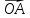, and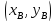the coordinates of the vector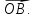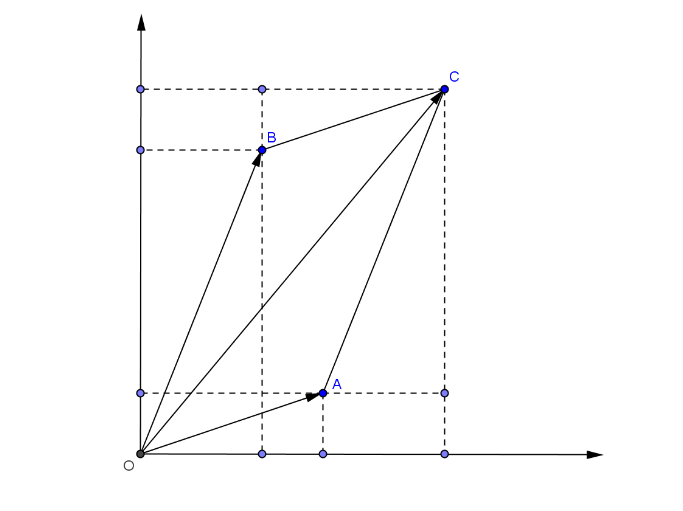a) Explain the geometric significance of the coordinatesof the point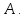(What do they represent in the diagram below? How are coordinates of a point constructed in general?)

b) Use congruent triangles and the properties of parallelograms and rectangles to prove thatif and only if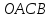is a parallelogram.

c) Let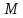be the midpoint of segment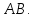Write a formula for the coordinates of M in terms of the coordinates of A and B. Which property of a parallelogram is useful in deriving this formula?